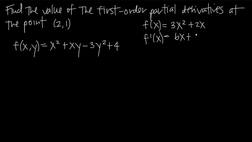PLAY PREVIEW

### Segments in this Video

#### First Order Partial Derivatives of a Function(01:41)

FREE PREVIEW

The chain rule is used to find the first order partial derivatives of a function in terms or two variables. Take the derivative of each variable separately when taking the derivative of a multivariable function.

#### Constructing Partial Derivatives(02:54)

We can take the partial derivative of a function with respect to a particular variable by treating all other variables as constants. In the context of derivatives, a "constant" is a value that does not change as the function changes.

#### Finding the Partial Derivative of the Function (01:51)

The presenter addresses the different types of derivatives when solving for a partial function.

#### Finding the Value of a Partial Derivative(00:31)

To evaluate a partial derivative at a particular point, plug in the point's coordinates for x and y in the equation for the partial derivative.

#### Credits(00:00)

Credits

For additional digital leasing and purchase options contact a media consultant at 800-257-5126
(press option 3) or sales@films.com.

# Partial derivatives in two variables

Part of the Series : Integral Calc: Calculus 3
 3-Year Streaming Price: \$49.95

Share

### Description

This video tutorial works through math problems/equations that address topics in Calculus 3, Partial Derivatives. This specific tutorial addresses Partial derivatives in two variables.

Length: 8 minutes

Item#: BVL275742Closed Captioned